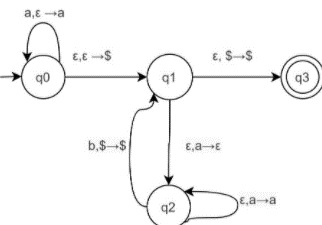# What is a Queue Automaton?

A queue automaton is like a push-down automaton (PDA) except that the stack is replaced by a queue.

• A queue is a tape allowing symbols to be written only on the left-hand end and read only at the right-hand end.

• Each write operation (we’ll call it a push) adds a symbol to the left-hand end of the queue and each read operation (we’ll call it a pull) reads and removes a symbol at the right-hand end.

• As with a PDA, the input is placed on a separate read-only input tape, and the head on the input tape can move only from left to right.

• The input tape contains a cell with a blank symbol following the input, so that the end of the input can be detected.

• A queue automaton accepts its input by entering a special accept state at any time.

## Formal Definition

A deterministic queue automaton (DQA) is defined as a seven tuple

(Q, Σ, Γ, δ, q0 , ⊥, F)

Where,

• Q is a set of states,

• Σ is an input alphabet,

• Γ is a finite set of queue symbols,

• q0 is a starting state,

• ⊥ is an empty queue symbol ⊥6= Γ,

• The transition function δ is defined by Q × Σ ∪ {λ} × (Γ × Γ) ∪ ({⊥} × {⊥}) → Q × Γ ∪ {λ} × {keep, remove}, where λ signifies empty symbol. It must never be used as an input symbol.

• F is a set of accepting states (F ⊆ Q).

## Example

Construct queue automata for language {anbn | n >= 0}.

For the given language the number of states present in the automata are 4,

Q={q0,q1,q2,q3},

Input alphabet ={a,b}

The queue automata is as follows −